# KVPY-SX 2019 Maths Paper with Solutions

KVPY (Kishore Vaigyanik Protsahan Yojana) is a national level scholarship program conducted to identify students with talent and aptitude for research. KVPY-SX 2019 Maths paper solutions are given on this page. BYJU’S provides step by step solutions that are prepared by subject experts. These solutions will help students to understand the marking scheme and pattern of the exam. Students are recommended to revise these solutions so that they can improve their speed and accuracy.

### KVPY SX 2019 - Maths

Question 1: The number of four-letter words that can be formed with letters a, b, c such that all three letters occur is:

1. (a) 30
2. (b) 36
3. (c) 81
4. (d) 256

Solution:

The 4 letter code will have a, b, c and a repeat letter from either a, b or c

The possible selections are

{a, a, b, c}, {b, b, a, c} and {c, c, a, b}

First selection is {a, a, b, c} = (arrangement of 4 words)/(repetition of a’s two times)

= 4!/2!

= 4×3×2/2

= 12

Second selection is {b, b, a, c} = (arrangement of 4 words)/(repetition of b’s two times)

= 4!/2!

= 4×3×2/2

= 12

Third selection is {c, c, a, b} = (arrangement of 4 words)/(repetition of c’s two times)

= 4!/2!

= 4×3×2/2

= 12

Number of four letter words that can be formed with letters a, b, c such that all three letters occur is = 12 + 12 + 12 = 36

Question 2: Let A = {θ ∈ R:{(1/3)sin θ+(2/3) cos θ}2= (1/3)sin2 θ +(2/3)cos2 θ} . Then

1. (a) A ⋂ [0, π] is an empty set
2. (b) A ⋂ [0, π] has exactly one point
3. (c) A ⋂ [0, π] has exactly two points
4. (d) A ⋂ [0, π] has more than two points

Solution:

Given A = {θ ∈ R: {(1/3)sin θ+(2/3) cos θ}2= (1/3)sin2 θ +(2/3)cos2 θ}

((1/3)sin θ+(2/3) cos θ)2 = (1/3)sin2 θ+(2/3)cos2 θ { since (A+B)2= A2+2AB+B2}

((1/9)sin2 θ+(4/9)sin θ cos θ+(4/9)+(4/9)cos2 θ = (1/3) sin2 θ+(2/3)cos2 θ

(2/9)sin 2θ = ((1/3)-(1/9))sin2 θ+((2/3)-(4/9)) cos2 θ {Since 2 sin A cos A = sin 2A}

(2/9)sin 2θ = (2/9) sin2 θ+(2/9) cos2 θ

(2/9)sin 2θ = (2/9)[sin2θ+cos2θ]

sin 2 θ = 1

sin 2 θ = sin 2(π/4)

2 θ = n π+(-1)n(π/4), n ∈ I

θ = (π/4) , θ ∈ [0, π]

A ⋂ [0, π] has exactly one point.

Question 3: The area of the region bounded by the lines x = 1, x = 2 and the curves x(y – ex) = sin x and 2xy = 2sin x + x3 is:

1. (a) e2 – e – 1/6
2. (b) e2 – e – 7/6
3. (c) e2 – e + 1/6
4. (d) e2 – e + 7/6

Solution:

Given curve

x(y – ex) = sin x

y = (sin x/x)+ex ...(1)

second curve

2xy = 2 sin x+x3 (given)

y = (sin x/x)+(x2/2) …(2)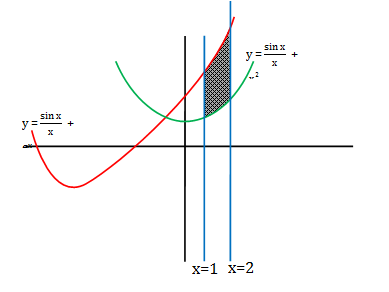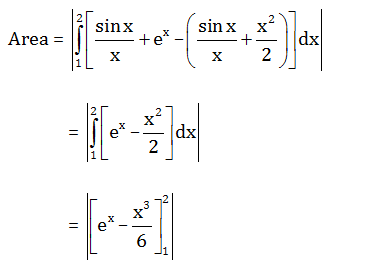= |[e2-(2)3/6 -e1+(1)3/6] |

= e2-e-(8/6)+(1/6)

Area = e2-e-7/6

Question 4: Let AB be a line segment with midpoint C, and D be the midpoint of AC. Let C1 be the circle with diameter AB, and C2 be the circle with diameter AC. Let E be a point on C1 such that EC is perpendicular to AB. Let F be a point on C2 such that DF is perpendicular to AB, and E and F lie on opposite sides of AB. Then the value of sin ∠ FEC is

1. (a) 1/√10
2. (b) 2/√10
3. (c) 1/√13
4. (d) 2/√13

Solution: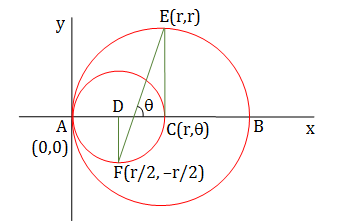Given that

∠ FEC = 90° –θ…………….. (1)

We are going to find out slope of FE

tanθ = slope of FE = (r-(-r/2))/(r-r/2)

tan θ = 3 = perpendicular/base⇒ cos θ = base/hypotenuse = 1/√10

⇒ sin (90° – θ) = 1/√10 {According to reduction formula}

Hence sin (∠ FEC) = 1//√10 {from equation (1)}

Question 5: The number of integers x satisfying -3x4 + det $\begin{bmatrix} 1 & x &x^{2} \\ 1 & x^{2} &x^{4} \\ 1 & x^{3} & x^{6} \end{bmatrix}=0$ is equal to

1. (a) 1
2. (b) 2
3. (c) 5
4. (d) 8

Solution:

Given -3x4 + det $\begin{bmatrix} 1 & x &x^{2} \\ 1 & x^{2} &x^{4} \\ 1 & x^{3} & x^{6} \end{bmatrix}=0$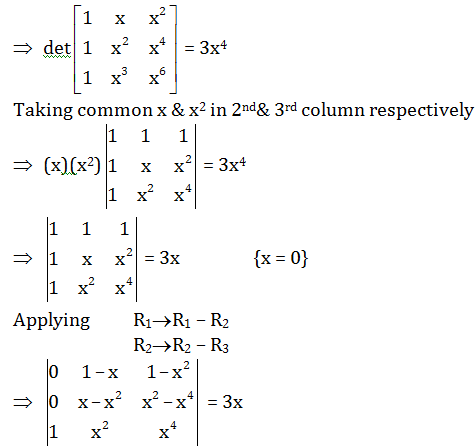⇒ x = 0 or x(1 – x)2(x2 – 1) = 3x

⇒ x = 0 or (1 – x)2(x2 – 1) = 3

⇒ x = 0 or x4–2x3+ 2x – 4 = 0

⇒ x = 0 or (x–2)(x3 + 2) = 0

Integer value are 0, 2.

Question 6: Let P be a non-zero polynomial such that P(1 + x) = P(1 – x) for all real x, and P(1) = 0. Let m be the largest integer such that (x – 1)m divides P(x) for all such P(x). Then m equals

1. (a) 1
2. (b) 2
3. (c) 3
4. (d) 4

Solution:

P(x) is non-zero polynomial and P(1 + x) = P(1 – x) for all x

Differentiate with respect to x

P’(1 + x) = (–1)P’(1 – x)

⇒ P’(1 + x) = –P’(1 – x)

Put x = 0

⇒ P’(1) = –P’(1)

⇒ P’(1) + P’(1) = 0

⇒ 2P’(1) = 0

⇒ P’(1) = 0

and P(1) = 0

⇒ P(x) touches the x-axis at x = 1

⇒ P(x) = (x – 1)2 Q(x)

⇒ m = 2 such that (x – 1)m divides P(x) for all such P(x).

Question 7: Let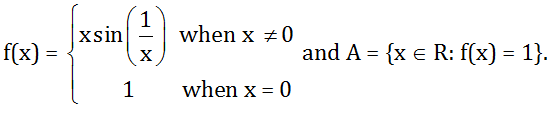Then A has

1. (a) exactly one element
2. (b) exactly two element
3. (c) exactly three element
4. (d) infinitely many elements

Solution:

Given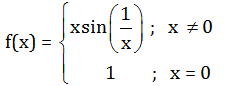A = {x ∈ R: f(x) = 1}

f(x) = 1 for x = 0

for x ≠ 0,

f(x) = 1

⇒ x sin 1/x = 1

⇒ sin 1/x = 1/x

⇒ sin θ = θ which is true only when θ = 0

As θ ≠ 0 so it is not possible.

Question 8: Let S be a subset of the plane defined by S = {(x, y); |x| + 2|y| = 1}. Then the radius of the smallest circle with centre at the origin and having non-empty intersection with S is

1. (a) 1/5
2. (b) 1/√5
3. (c) 1/2
4. (d) 2/√5

Solution:

Given

S = {(x, y); |x| + 2|y| = 1}

So |x| + 2|y| = 1

Equations are

x + 2y = 1 ……………. (1)

x – 2y = 1 ……………. (2)

–x + 2y = 1 ……………. (3)

–x – 2y = 1 ……………. (4)

Find intersecting point equation (1) & (2)

x + 2y = 1

x – 2y = 1

2x = 2

x = 1

So y = 0 {from equation (1)}

Intersecting point of equations (1) & (2) is (1, 0).

Similarly,

Find intersecting point equation (1) & (3)

x + 2y = 1

–x + 2y = 1

4y = 2

y = 1/2

x = 0 {from equation (1)}

Intersecting point of equations (1) & (3) is (0, 1/2).

Find intersecting point equation (2) & (4)

x – 2y = 1

–x – 2y = 1

–4y = 2

y = –1/2

x = 0 {from equation (2)}

Intersecting point of equations (2) & (4) is (0, –1/2).

Find intersecting point equation (3) & (4)

–x + 2y = 1

–x – 2y = 1

–2x = 2

x = –1

So y = 0 {from equation (3)}

Intersecting point of equations (3) & (4) is (–1, 0)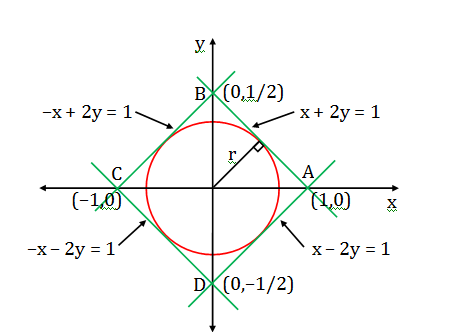Length of the perpendicular from a point (x1, y1) to a line ax + by = c is

$d=\left | \frac{ax_{1}+by_{1}-c}{\sqrt{a^{2}+b^{2}}} \right |$

According to question minimum radius is the distance between point (0, 0) and line x + 2y = 1.

So, Minimum radius = |(0+2×0-1)/ √(12+22)|

= |-1/ √(1+4)|

= 1/√5

Question 9: The number of solutions of the equation sin (9x) + sin (3x) = 0 in the closed interval [0, 2 π] is

1. (a) 7
2. (b) 13
3. (c) 19
4. (d) 25

Solution:

Given

sin(9x) + sin(3x) = 0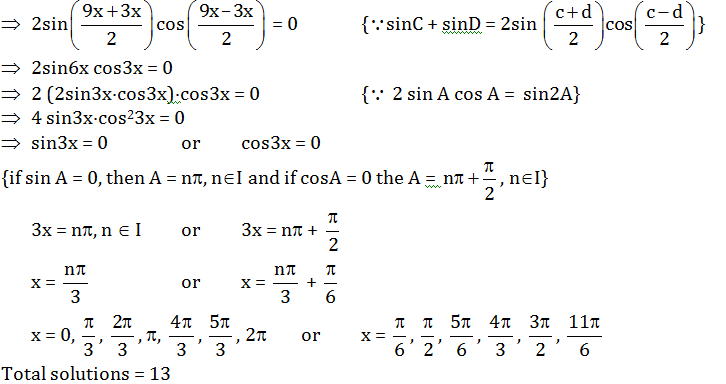Question 10: Among all the parallelograms whose diagonals are 10 and 4, the one having maximum area has its perimeter lying in the interval

1. (a) (19, 20]
2. (b) (20, 21]
3. (c) (21, 22]
4. (d) (22, 23]

Solution:

Area of parallelogram = (1/2)d1d2sin φ

Where d1 and d2 is the diagonal of the parallelogram.

Maximum area of parallelogram = ½ d1d2 {since where φ =π/2 }

It is a rhombus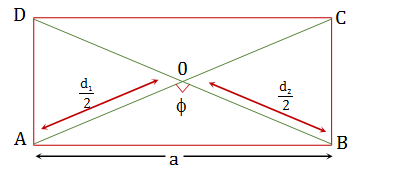ΔAOB is a right a triangle so apply Pythagoras theorem

So, a2 = (d1/2)2

Side length (a) = √((d1/2)2+ (d2/2)2)

⇒ (a) = √((10/2)2+ (4/2)2)

⇒ (a) = √((5)2+ (2)2)

⇒ (a) = √(25+4)

⇒ (a) = √29

Perimeter = 4a

= 4(√29 ) ∈ [21, 22)

Question 11: The number of ordered pairs (a, b) of positive integers such that (2a-1)/b and (2b-1)/a are both integers is

1. (a) 1
2. (b) 2
3. (c) 3
4. (d) more than 3

Solution:

Given (2a-1)/b and (2b-1)/a are both integers.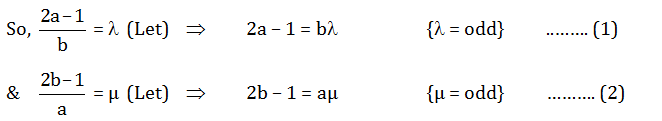From equation (1)

⇒ 4a – 2 = 2b λ

⇒ 4a – 2 = λ (µa + 1) {From equation (2)}

⇒ (4 – λ µ)a = λ + 2

Since multiplication of λ and µ lie between only 1 & 3 because a and b are positive integer.

So 1 ≤µ ≤ 3

In equation (2) put λ = 1, µ = 1

(4 – 1) a = 1 + 2 ⇒ a = 1, b = 1

Similarly,

λ = 1, µ = 3 ⇒ a = 3, b = 5

λ = 3, µ = 1 ⇒ a = 5, b = 3

So, total set = 3

(1, 1), (3, 5), (5, 3)

Question 12: Let z = x + iy and w = u + iv be complex numbers on the unit circle such that z2 + w2 = 1. Then the number of ordered pairs (z, w) is

1. (a) 0
2. (b) 4
3. (c) 8
4. (d) infinite

Solution:

Let

z = e = cos α + i sin α

w = e = cos β + i sin β

Since, z2 + w2 = 1

⇒ (cos α + i sin α)2 + (cos β + i sin β)2 = 1 {(A + iB)2 = A2–B2 + 2iAB}

⇒ cos2α – sin2α + 2i sinαcosα + cos2β – sin2β + 2i sinβcosβ = 1

⇒ (cos2 α + cos2 β) + i(sin2 α + sin2 β ) = 1{cos2A = cos2A – sin2A, sin2A = 2sinA cosA}

Comparing real and imaginary part

So cos2 α + cos2 β = 1 and sin2 α + sin2 β = 0

2cos(α + β)cos(α -β) = 1

And sin2 α = –sin2 β

Squaring both side

sin22 α = sin22 β

1 – cos22 α = 1 – cos22 β

cos22 α = cos22 β

Taking square root both side

cos2 α = ±cos2 β

cos2 α = –cos2 β

or cos2 α + cos2 β = 0 (cancelled)

If cos2 α = cos2 β

So 2cos2 α = 1

cos 2α = ½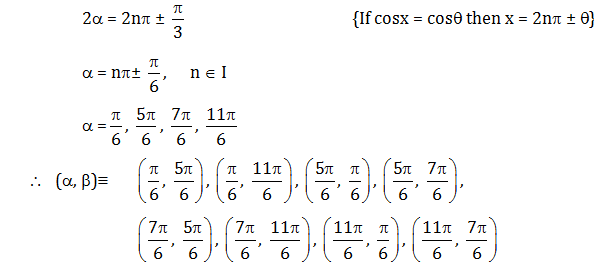Question 13: Let E denote the set of letters of the English alphabet, V = {a, e, i, o, u}, and C be the complement of V in E. Then, the number of four-letter words (where repetitions of letters are allowed) having at least one letter from V and at least one letter from C is

1. (a) 261870
2. (b) 314160
3. (c) 425880
4. (d) 851760

Solution:

‘V’ denotes vowels and ‘C’ denotes consonants.

Total alphabet words = 26

Total vowel in alphabet = 5

Total consonant in alphabet = 21

Total 4 letter words = (26)4

Number of 4 letter words which contains only vowels = (5)4

Number of 4 letter words which contains only consonant = (21)4

Number of 4 letter words which contains atleast one vowel and atleast one consonants:

(26)4 – (21)4 – (5)4 = 261870

Question 14: Let σ1, σ2, σ3 be planes passing through the origin. Assume that σ1 is perpendicular to the vector (1, 1, 1), σ2 is perpendicular to a vector (a, b, c), and σ3 is perpendicular to the vector (a2, b2, c2). What are all the positive values of a, b, and c so that σ1⋂ σ2 ⋂σ3 is a single point?

1. (a) Any positive value of a, b, and c other than 1
2. (b) Any positive value of a, b, and c where either a ≠ b, b ≠ c or a ≠ c
3. (c) Any three distinct positive values of a, b, and c
4. (d) There exist no such positive real numbers a, b, and c

Solution:

σ1 is perpendicular to $\hat{i}+\hat{j}+\hat{k}$

σ2 is perpendicular to $a\hat{i}+b\hat{j}+c\hat{k}$

σ3 is perpendicular to $a^{2}\hat{i}+b^{2}\hat{j}+c^{2}\hat{k}$

since σ1 , σ2 and σ3 planes are passing through the origin

Hence the planes are

σ1: x + y + z = 0

σ2: ax + by + cz = 0

σ3: a2x + b2y + c2z = 0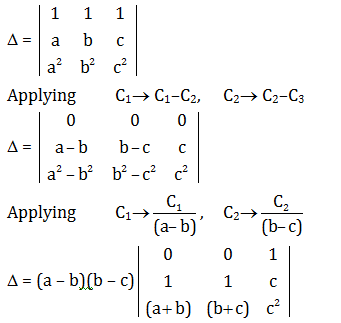Δ = (a – b)(b – c)(c – a)

For unique solution, Δ ≠ 0

⇒ (a – b)(b – c)(c – a) ≠ 0

Hence a ≠ b, b ≠ c, c ≠ a

Question 15: Ravi and Rashmi are each holding 2 red cards and 2 black cards (all four red and all four black cards are identical). Ravi picks a card at random from Rashmi, and then Rashmi picks a card at random from Ravi. This process is repeated a second time. Let p be the probability that both have all 4 cards of the same colour. Then p satisfies

1. (a) p≤ 5%
2. (b) 5% < p ≤10%
3. (c) 10% < p ≤ 15%
4. (d) 15% < p

Solution: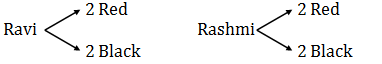If Ravi withdraw red cards from Rashmi, then Rashmi withdraw black card from Ravi and this process repeat again, vice-versa. If Ravi withdraw black card from Rashmi

P = 2(2/4)(2/5)(1/4)(1/5)

P = 2/100

P = 2%

Question 16: Let A1, A2 and A3 be the regions on R2 defined by

A1 = {(x, y); x ≥ 0, y ≥0, 2x + 2y – x2 – y2> 1 > x + y},

A2 = {(x, y); x ≥ 0, y ≥0, x + y > 1 > x2 + y2},

A3 = {(x, y); x ≥ 0, y ≥0, x + y > 1 > x3 + y3},

Denote by |A1|, |A2| and |A3| the areas of the regions A1, A2, and A3 respectively. Then

1. (a) |A1| > |A2| > |A3|
2. (b) |A1| > |A3| > |A2|
3. (c) |A1| = |A2| < |A3|
4. (d) |A1| = |A3| > |A2|

Solution:

Given

A1 = {(x, y); x ≥ 0, y ≥0, 2x + 2y – x2 – y2> 1 > x + y}

A2 = {(x, y); x ≥ 0, y ≥0, x + y > 1 > x2 + y2}

A3 = {(x, y); x ≥ 0, y ≥0, x + y > 1 > x3 + y3}

For A1: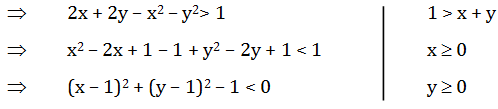Find intersecting point of circle & line

⇒ (x – 1)2 + (1 – x – 1)2 = 1 {Line x + y = 1 put in circle equation.}

⇒ x2 – 2x + 1 + x2 = 1

⇒ 2x2 – 2x = 0

⇒ x (x –1) = 0

⇒ x = 0, 1

Hence y = 1, 0

(0, 1) and (1, 0)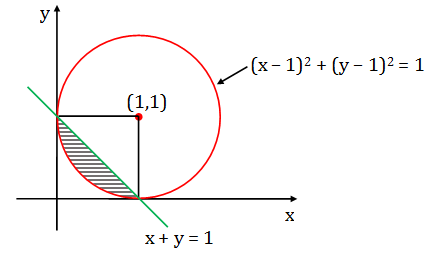Area of A1 = (1/4)of area of circle- area of triangle

= π(1)2/4 -(1/2)(1)(1)

= (π/4)-(1/2)

For A2:

A2 = {(x, y); x ≥ 0, y ≥0, x + y > 1 > x2 + y2},

⇒ 1 > x2 + y2, x + y > 1, x ≥ 0, y ≥ 0

Find intersecting point of circle & line

⇒ (x)2 + (1 – x)2 = 1 {Line x + y = 1 put in circle equation.}

⇒ x2 + 1– 2x + x2 = 1

⇒ 2x2 – 2x = 0

⇒ x (x –1) = 0

⇒ x = 0, 1

So y = 1, 0

(0, 1) and (1, 0)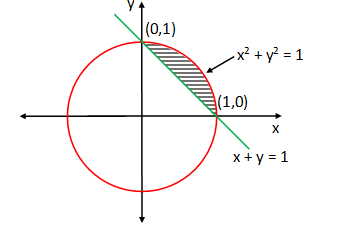Area of A2= 1/4 of area of circle – Area of triangle

= π(1)2/4 – 1/2(1)(1)

A2 = π/4 – 1/2

For A3:

A3 = {(x, y); x ≥ 0, y ≥0, x + y > 1 > x3 + y3}

⇒ x + y > 1, x3 + y3< 1, x ≥ 0, y ≥ 0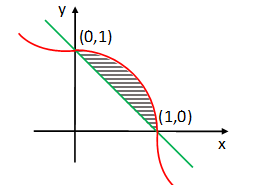Hence |A3| > |A2| = |A1|

Question 17: Let f : R-> R be a continuous function such that f (x2) = f (x3) for all x ∈ R. Consider the following statements.

(I) f is an odd function

(II) f is an even function

(III) f is differentiable everywhere.

Then

1. (a) I is true and III is false
2. (b) II is true and III is false
3. (c)both I and III are true
4. (d) both II and III are true

Solution:

f: R -> R be continuous function such that

f(x2) = f(x3) …(1)for all x belongs to R

Put x = –x

f(x2) = f(–x3)

From equation (1) we have f(x3) = f(–x3)

Put x3 = t we have f(t) = f(–t)

So f(x) is an even function.

(ii) Now take x3 = t

Then f(t2/3) = f(t)

Put t = t2/3

Then f((t 2/3)2) = f(t)

f(t) = f(t 2/3) = f((t 2/3)2) = f((t 2/3)3) = ……….. = f((t 2/3) n)

This is true for all t belongs to R and any n belongs to I

Hence if we take n -> ∞, (2/3)n -> 0

then f(t) = f(t0) = 1

So f(x) is a constant function, hence it is differentiable everywhere.

Question 18: Suppose a continuous function f : [0, ∞) -> R satisfies $f(x)=2\int_{0}^{x}tf(t)dt+1$ for all x $\geq$ 0. Then f(1) equals:

1. (a) e
2. (b) e2
3. (c) e4
4. (d) e6

Solution:

Given

f: [0, ∞)-> R

f(x) = $2\int_{0}^{x}tf(t)dt+1$, for all x >0

Differentiate with respect to x

So f '(x) = 2.x.f(x)

f'(x)/f(x) = 2x

Integrate both sides

$\int \frac{f'(x)}{f(x)}= \int 2x\: dx$

Ln f(x) = x2 + c

f(x) = $e^{x^{2}}+c$

Since f(0) = 1

put x = 0, y = 1

f(0) = e1 + c

c = 0

So f(x) = $e^{x^{2}}+0$

f(x) = $e^{x^{2}}$

f(1) = e

Question 19: Let a > 0, a ≠ 1. Then the set S of all positive real numbers b satisfying (1 + a2) (1+ b2) = 4ab is

1. (a) an empty set
2. (b) a singleton set
3. (c) a finite set containing more than one element
4. (d) (0, ∞)

Solution:

a> 0, a ≠ 1

(1 + a2)(1 + b2) = 4ab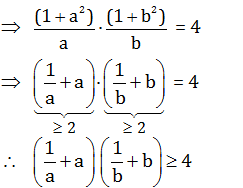Equality holds true when a = b = 1

But it is given in the question that a ≠ 1

Hence, there are no values of b.

Question 20: Let f : R -> R be a function defined by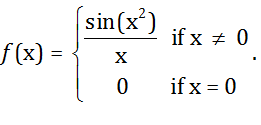Then, at x = 0, f is:

1. (a) Not continuous
2. (b) Continuous but not differentiable
3. (c) Differentiable and the derivative is not continuous
4. (d) Differentiable and the derivative is continuous

Solution:

f : R -> R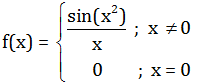Check continuity: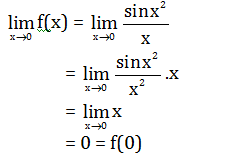Hence, f(x) is continuous at x = 0

Check differentiability: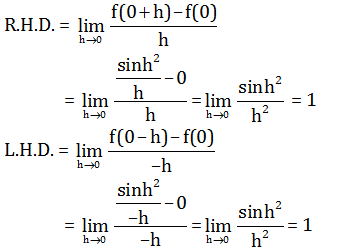R.H.D. = L.H.D.

f(x) is differentiable at x = 0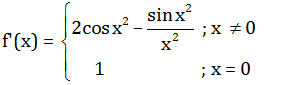For continuity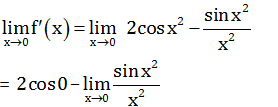= 2 – 1 = 1

Derivative is continuous at x = 0.

Question 21: The points C and D on a semicircle with AB as diameter are such that AC = 1, CD = 2, and DB = 3. Then the length of AB lies in the interval

1. (a) [4, 4.1)
2. (b) [4.1, 4.2)
3. (c) [4.2, 4.3)
4. (d) [4.3, ∞ )

Solution: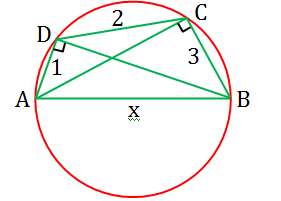Let AB = x

ΔABC,

(AB)2 = (AC)2 + (BC)2 { since ΔABC is a right angle triangle}

BC = √[x2-1]

ΔABD,

(AB)2 = (AD)2 + (BD)2 {since ΔABD is a right angle triangle}

Apply Ptolemy theorem, we get

AB × CD + AC × BD = AD × BC

2x + 3 = √[x2-1] √[x2-9]

On squaring we get

(2x + 3)2 = [√[x2-1] √[x2-9]]2

4x2 + 12x + 9 = (x2 – 1)(x2 – 9) {(A + B)2 = A2 + B2 + 2AB}

4x2 + 12x + 9 = x4 – 10x2 + 9

x4 – 14x2 – 12x = 0

x3 – 14x – 12 = 0

f(x) = x3 – 14x – 12

f(4.1) = (4.1)3 – 14(4.1) – 12 = –0.479

f(4.2) = (4.2)3 – 14(4.2) – 12 = 3.288

f(4.1).f(4.2) < 0

As f(x) is a continuous function therefore one root of f(x) lies in [4.1, 4.2) i.e. length of AB lies in this interval.

Question 22: Let ABC be a triangle and let D be the midpoint of BC. Suppose cot(∠CAD) : cot(∠BAD) = 2 : 1. If G is the centroid of triangle ABC, then the measure of ∠ BGA is

1. (a) 900
2. (b) 1050
3. (c) 1200
4. (d) 1350

Solution: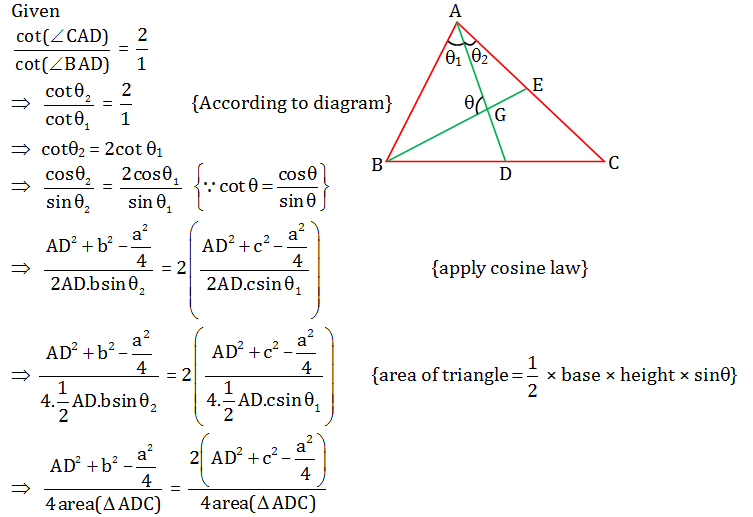(Using the formula of length of median, AD = 1/2 √(2b2+2c2-a2)

(a2/4)+b2 = 2c2+(1/4)(2b2+2c2-a2)

a2/2+ b2/2= 5c2/2

a2+ b2= 5c2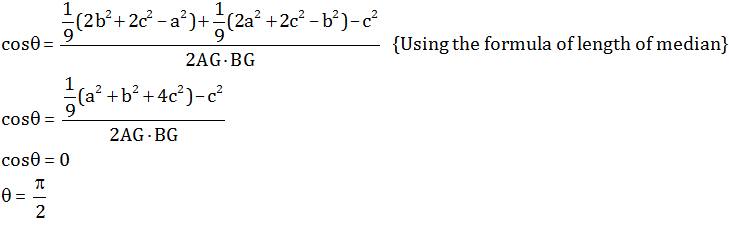Question 23: Let f(x) = x6 – 2x5 + x3 + x2 – x – 1 and g(x) = x4 – x3 – x2 – 1 be two polynomials. Let a, b, c and d be the roots of g(x) = 0. Then the value of f(a) + f(b) + f(c) + f(d) is

1. (a) -5
2. (b) 0
3. (c) 4
4. (d) 5

Solution:

Given

g(x) = x4 – x3 – x2 – 1 = 0

f(x) = x6 – 2x5 + x3 + x2 – x – 1

since a, b, c, d are roots of x4 – x3 – x2 – 1 = 0

Hence ∑a = 1

∑ab = -1

Now,

f(x) = x6 – 2x5 + x3 + x2 – x – 1

f(x) = x2(x4 – x3 – x2 – 1) – x(x4 – x3 – x2 – 1) + (2x2 – 2x – 1)

f(x) = (x2 – x) (x4 – x3 – x2 – 1) + (2x2 – 2x – 1)

f(x) = 2x2 – 2x – 1 {since x4 – x3 – x2 – 1 = 0}

Now,

⇒f(a) + f(b) + f(c) + f(d)

⇒2a2 – 2a – 1 + 2b2 – 2b – 1 + 2c2 – 2c – 1 + 2d2 – 2d – 1

⇒2[a2 + b2 + c2 + d2] – 2[a + b + c + d] – 4

⇒2[(a + b + c + d)2 – 2(ab + bc + cd + ac + bd)] – 2[a + b + c + d] – 4

⇒2[1 – 2(–1)] – 2(1) – 4 = 0

Question 24: Let $\vec{a}= \hat{i}+\hat{j}+\hat{k}$ , $\vec{b}= 2\hat{i}+2\hat{j}+\hat{k}$ and $\vec{c}= 5\hat{i}+\hat{j}-\hat{k}$ be three vectors. The area of the region formed by the set of points whose position vectors $\vec{r}$ satisfy the equations $\vec{r}.\vec{a}=5$ and $\left |\vec{r}-\vec{b} \right |+\left |\vec{r}-\vec{c} \right |=4$ is closest to the integer

1. (a) 4
2. (b) 9
3. (c) 14
4. (d) 19

Solution:

Given

$\vec{a}= \hat{i}+\hat{j}+\hat{k}$

$\vec{b}= 2\hat{i}+2\hat{j}+\hat{k}$

$\vec{c}= 5\hat{i}+\hat{j}-\hat{k}$

$\vec{r}.\vec{a}=5$

⇒ x+y+z = 5

$\left |\vec{r}-\vec{b} \right |+\left |\vec{r}-\vec{c} \right |=4$

Sum of distances of a point $\vec{r}$ from two fixed points with position vector $\vec{b}\: and\: \vec{c}$ is constant.

$\left | \vec{b}- \vec{c}\right |=\left | 2\hat{i}+2\hat{j}+\hat{k}-5\hat{i} -\hat{j}+\hat{k}\right |$

= $\left | -3\hat{i}+\hat{j}+2\hat{k}\right |$

= $\sqrt{(3^{2})+1^{2}+2^{2}}$

= $\sqrt{14}$

With $\vec{b}$and $\vec{c}$ lie on the plane x + y + z = 5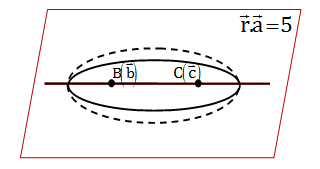Area in the plane constitutes an ellipse

Distance between $\vec{b}$ and $\vec{c}$ = 2 × (semi major axis) × e

= $\sqrt{14}$

2ae = $\sqrt{14}$

2a = 4

So 4e = $\sqrt{14}$

e = $\frac{\sqrt{14}}{4}$

Eccentricity (e) = √(1-b2 /a2)

(√14/4)2 = 1-b2/a2

b2 = ½

area of ellipse = πab

= π(2)1/√2

= √2 π

Question 25: The number of solutions to sin(π sin2(θ)) + sin(π cos2(θ)) = 2cos (π/2 cos θ ) satisfying 0 ≤ θ ≤ 2 π is

1. (a) 1
2. (b) 2
3. (c) 4
4. (d) 7

Solution:

Given sin(π sin2(θ)) + sin(π cos2(θ)) = 2cos (π/2 cos θ )

{sin C+sin D = 2 sin ((C+D)/2 )cos((C-D)/2)}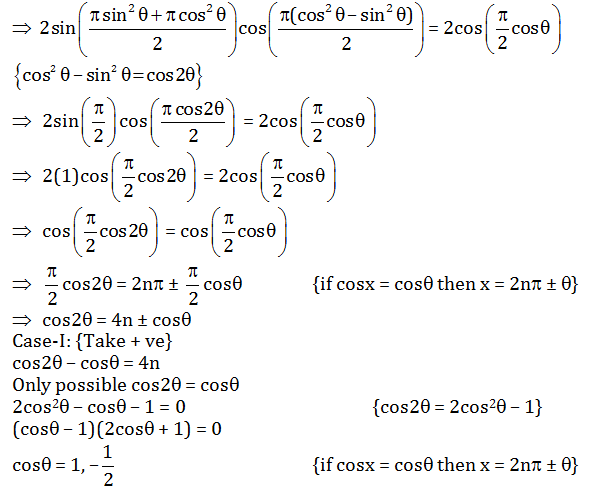θ = 2n π, 2n π ±2n π/3

θ belongs to {0, 2 π, 2 π/3, 4 π/3}

Case-II: {Take – ve}

cos2 θ + cos θ = 4n

Only possible cos2 θ + cos θ = 0 {cos2 θ = 2cos2 θ – 1}

2cos2 θ + cos θ – 1 = 0

(cos θ + 1)(2cos θ – 1) = 0

cos θ = –1, 1/2

θ = (2n + 1) π, 2n π ± π/3

θ belongs to { π, π/3, 5 π/3}

Total solutions is 7.

Question 26: Let J = $\int_{0}^{1}\frac{x}{1+x^{8}}dx$

Consider the following assertions:

I. J > 1/4

II. J < π/8

Then

1. (a) Only I is true
2. (b) only II is true
3. (c) both I and II are true
4. (d) neither I nor II is true

Solution:

Given J = $\int_{0}^{1}\frac{x}{1+x^{8}}dx$

Now,1 + x8< 2 {Limit 0 to 1}

1/(1+x8)>1/2 ⇒ x/(1+x8)>x/2

Apply integration both sides

$\int_{0}^{1}\frac{x}{1+x^{8}}dx>\int_{0}^{1}\frac{x}{2}$

$J>\left [ \frac{x^{2}}{4} \right ]^{1}_{0}$

J>1/4

Statement I is true.

Now,

1 + x4> 1 + x8

1/(1+x4)<1/(1+x8)

x/1+x8>x/(1+x4)

Apply integration on both sides

$\int_{0}^{1}\frac{x}{1+x^{8}}dx> \int_{0}^{1}\frac{x}{1+x^{4}}dx$

$J> \int_{0}^{1}\frac{x}{1+x^{4}}dx$

Put x2 = t

2xdx = dt

$J> \frac{1}{2}\int_{0}^{1}\frac{dt}{1+t^{2}}$

$J> \frac{1}{2}[\tan t]^{1}_{0}$

J>(1/2) π/4

J> π/8

Question 27: Let f: (–1, 1) -> R be a differentiable function satisfying (f '(x))4 = 16(f(x))2 for all x belongs to (–1, 1), f(0) = 0. The number of such function is

1. (a) 2
2. (b) 3
3. (c) 4
4. (d) more than 4

Solution:

Given

(f '(x))4 = 16(f(x))2

Taking square root both side.

(f '(x))2 = ±4(f(x))

Case-I:

If f(x) > 0

(f '(x))2 = 4(f(x))

Taking square root both side

f '(x) = ±2√f(x)

f '(x) = 2√f(x)

f '(x)/ √f(x) = 2

Integrate both side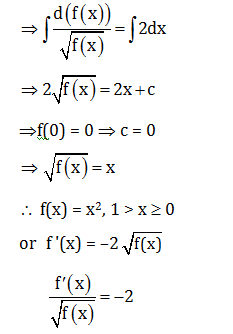Integrate both side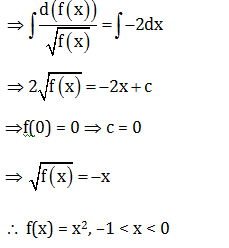Case-II:

If f(x) < 0

(f '(x))2 = –4(f(x))

f '(x) = ±2 √-f(x)

f '(x) = 2√-f(x) or f '(x) = –2√-f(x)

Similarly f(x) = –x2, –1 < x < 0 or f(x) = –x2, 1 > x > 0

Case-III:

Also, one singular solution of given differential equation is

f(x) = 0, –1 < x < 1

Hence, functions can be

f(x) = x2, –1 < x < 1

f(x) = –x2, –1 < x < 1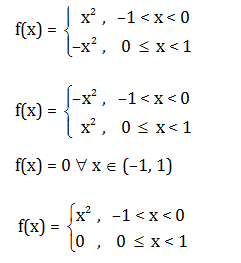More functions are also possible.

Question 28: For x ∈ R, let f(x) = |sin x| and g(x) = $\int_{0}^{x}f(t)dt$ . Let p(x) = g(x) – (2/ π)x. Then

1. (a) p(x + π) = p(x) for all x
2. (b) p(x + π) ≠ p(x) for at least one but finitely many x
3. (c) p(x + π) ≠ (x) for infinitely many x
4. (d) p is a one-one function

Solution:

Given

f(x) = |sin x| …(1)

g(x) = $\int_{0}^{x}f(t)dt$ …(2)

p(x) = g(x) – (2/π) x …(3)

From equation (2)

g(x) =$\int_{0}^{x}f(t)dt$

Put x-> x+π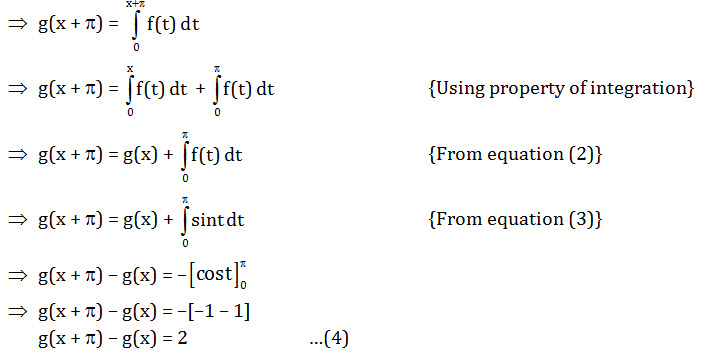From equation (3)

p(x) = g(x) – (2/π)x

Put x -> x + π

p(x + π) = g(x + π) – 2/ π (x + π)

p(x + π) = g(x) + 2 – 2x/ π – 2 {From equation (4)}

p(x + π) = g(x) – 2x/ π …(5)

eq.(5) – eq.(3)

p(x + π) – p(x) = g(x + π) – g(x) – 2

p(x + π) – p(x) = 2 – 2

p(x + π) = p(x) for all x.

Question 29: Let A be the set of vectors $\vec{a}$ = (a1, a2, a3) satisfying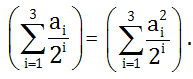Then

1. (a) A is empty
2. (b) A contains exactly one element
3. (c) A has 6 elements
4. (d) A has infinitely many elements

Solution:

Given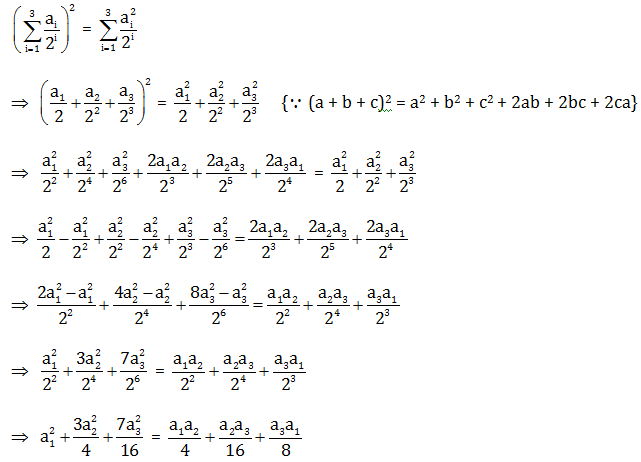a12+(3a22/4)+(7a32/16) = a1a2+ a2a3 /4+ a3a1/2

(16 a12+12a22+7a32)/16 = (4a1a2+a2a3+2a3a1)/4

16 a12+12a22+7a32 = 4(4a1a2+a2a3+2a3a1)

16 a12+12a22+7a32 - 16a1a2-4a2a3-8a3a1 = 0

8 a12- 16a1a2 +8a22 +8a12-8a1a3+2a32+4a2 2-4a2a1+a32+4a32 = 0

(2√2a1-2√2a2)2+(2√2a1-√2a3)2+(2a2-a3)2+4a32 = 0

Only possible when a1 = a2 = a3 = 0

So only one element in the set.

Question 30: Let f : [0, 1] -> [0, 1] be a continuous function such that x2 + (f(x))2 ≤ 1 for all

x ∈ [0, 1] and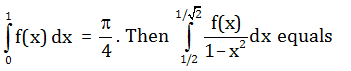1. (a) π/12
2. (b) π/15
3. (c) (√2-1) π /2
4. (d) π/10

Solution:

Given

x2 + (f(x))2 ≤ 1

Let y = f(x)

x2 + y2 ≤ 1 for all x belongs to [0, 1]

y2 ≤ 1 –x2

y = λ√(1-x2)

Apply integration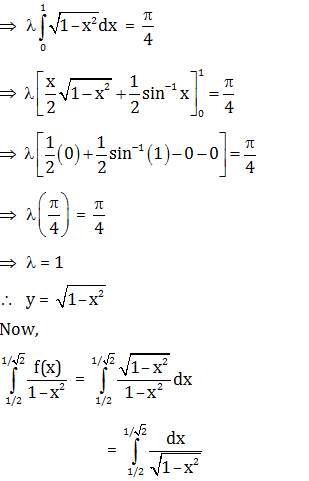= $\left [ \sin ^{-1}x \right ]_{\frac{1}{2}}^{\frac{1}{\sqrt{2}}}$

= $\left [ \sin ^{-1}\frac{1}{\sqrt{2}} -\sin ^{-1}\frac{1}{2}\right ]$

= π/4- π/6

= π/12

### KVPY-SX 2019 Maths Paper with Solutions